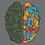# $\text{Original \& Alternating}$

\begin{aligned} 1 + \dfrac12 + \dfrac13 + \dfrac14 + \dfrac15 + \dfrac16 + \dfrac17 + \dfrac18 + \dots &= \infty \\ 1 - \dfrac12 + \dfrac13 - \dfrac14 + \dfrac15 - \dfrac16 + \dfrac17 - \dfrac18 + \dots &= \ln2 \\ \\ 1 + \dfrac13 + \dfrac15 + \dfrac17 + \dfrac19 + \dfrac{1}{11} + \dfrac1{13} + \dfrac1{15} + \dots &= \infty \\ 1 - \dfrac13 + \dfrac15 - \dfrac17 + \dfrac19 - \dfrac{1}{11} + \dfrac1{13} - \dfrac1{15} + \dots &= \dfrac{\pi}{4} \\ \\ 1 + \dfrac12 + \dfrac14 + \dfrac18 + \dfrac1{16} + \dfrac{1}{32} + \dfrac1{64} + \dfrac1{128} + \dots &= 2 \\ 1 - \dfrac12 + \dfrac14 - \dfrac18 + \dfrac1{16} - \dfrac{1}{32} + \dfrac1{64} - \dfrac1{128} + \dots &= \dfrac23 \\ \\ 1 + \frac1{2^2} + \dfrac1{3^2} + \dfrac1{4^2} + \dfrac1{5^2} + \dfrac1{6^2} + \dfrac1{7^2} + \dfrac1{8^2} + \dots &= \dfrac{\pi^2}{6} \\ 1 - \frac1{2^2} + \dfrac1{3^2} - \dfrac1{4^2} + \dfrac1{5^2} - \dfrac1{6^2} + \dfrac1{7^2} - \dfrac1{8^2} + \dots &= \dfrac{\pi^2}{12} \\ \\ 1 + \frac1{2!} + \dfrac1{3!} + \dfrac1{4!} + \dfrac1{5!} + \dfrac1{6!} + \dfrac1{7!} + \dfrac1{8!} + \dots &= e - 1 \\ 1 - \frac1{2!} + \dfrac1{3!} - \dfrac1{4!} + \dfrac1{5!} - \dfrac1{6!} + \dfrac1{7!} - \dfrac1{8!} + \dots &= 1 - \dfrac1e \\ \\ 1 + \frac1{3!} + \dfrac1{5!} + \dfrac1{7!} + \dfrac1{9!} + \dfrac1{11!} + \dfrac1{13!} + \dfrac1{15!} + \dots &= \sinh1 \\ 1 - \frac1{3!} + \dfrac1{5!} - \dfrac1{7!} + \dfrac1{9!} - \dfrac1{11!} + \dfrac1{13!} - \dfrac1{15!} + \dots &= \sin1 \\ \\ 1 + \frac1{2!} + \dfrac1{4!} + \dfrac1{6!} + \dfrac1{8!} + \dfrac1{10!} + \dfrac1{12!} + \dfrac1{14!} + \dots &= \cosh1 \\ 1 - \frac1{2!} + \dfrac1{4!} - \dfrac1{6!} + \dfrac1{8!} - \dfrac1{10!} + \dfrac1{12!} - \dfrac1{14!} + \dots &= \cos1 \\ \\ 1 + 2 + 3 + 4 + 5 + 6 + 7 + 8 + \dots &= \dfrac{-1}{12} \\ 1 - 2 + 3 - 4 + 5 - 6 + 7 - 8 + \dots &= \dfrac{1}{4} \\ \end{aligned}

$\text{Source: blackpen}\textcolor{#D61F06}{redpen}$1 year, 2 months ago

This discussion board is a place to discuss our Daily Challenges and the math and science related to those challenges. Explanations are more than just a solution — they should explain the steps and thinking strategies that you used to obtain the solution. Comments should further the discussion of math and science.

When posting on Brilliant:

• Use the emojis to react to an explanation, whether you're congratulating a job well done , or just really confused .
• Ask specific questions about the challenge or the steps in somebody's explanation. Well-posed questions can add a lot to the discussion, but posting "I don't understand!" doesn't help anyone.
• Try to contribute something new to the discussion, whether it is an extension, generalization or other idea related to the challenge.

MarkdownAppears as
*italics* or _italics_ italics
**bold** or __bold__ bold
- bulleted- list
• bulleted
• list
1. numbered2. list
1. numbered
2. list
Note: you must add a full line of space before and after lists for them to show up correctly
paragraph 1paragraph 2

paragraph 1

paragraph 2

[example link](https://brilliant.org)example link
> This is a quote
This is a quote
    # I indented these lines
# 4 spaces, and now they show
# up as a code block.

print "hello world"
# I indented these lines
# 4 spaces, and now they show
# up as a code block.

print "hello world"
MathAppears as
Remember to wrap math in $$ ... $$ or $ ... $ to ensure proper formatting.
2 \times 3 $2 \times 3$
2^{34} $2^{34}$
a_{i-1} $a_{i-1}$
\frac{2}{3} $\frac{2}{3}$
\sqrt{2} $\sqrt{2}$
\sum_{i=1}^3 $\sum_{i=1}^3$
\sin \theta $\sin \theta$
\boxed{123} $\boxed{123}$

Sort by:

- 1 year, 2 months ago

Glad you liked it @Yajat Shamji

- 1 year, 2 months ago

If you sourced BlackpenRedPen, he mentions that the second-last series has value $\dfrac{-1}{8}$ and not $\dfrac{-1}{12}$, although there can be many more values according to algebraic manipulations. Afterall $\infty= \infty+ 1$

- 1 year, 2 months ago

I used Ramanujan's summation.

- 1 year, 2 months ago